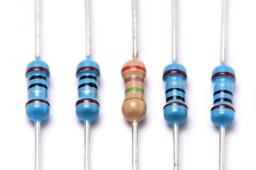# Two conductors

Two conductors have resistance Rs=5 ohms when connected in series and Rp=1.2 ohms when connected in parallel. Calculate the value of the resistors.

R1 =  3 Ω
R2 =  2 Ω

### Step-by-step explanation:Did you find an error or inaccuracy? Feel free to write us. Thank you!

Tips for related online calculators
Are you looking for help with calculating roots of a quadratic equation?
Do you have a linear equation or system of equations and looking for its solution? Or do you have a quadratic equation?

#### Grade of the word problem:

We encourage you to watch this tutorial video on this math problem: